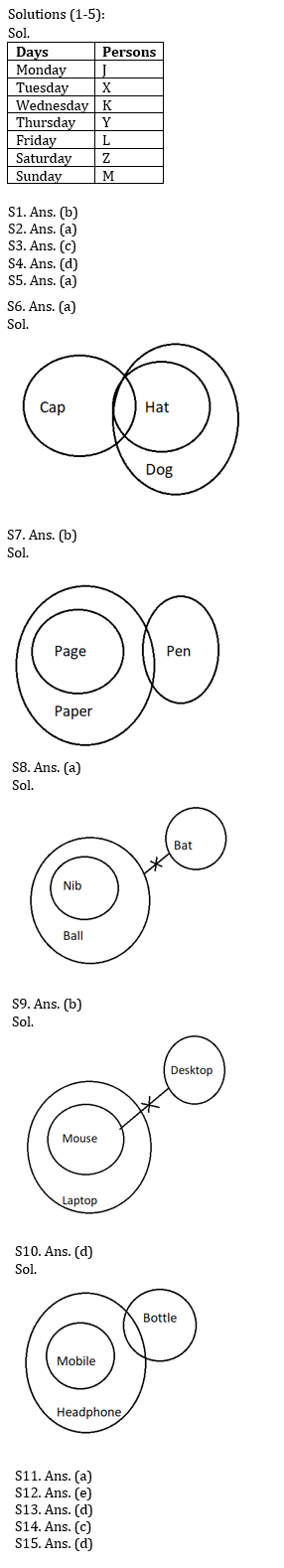Latest Banking jobs   »   Reasoning Ability Quiz For ESIC- UDC,...

# Reasoning Ability Quiz For ESIC- UDC, Steno, MTS Prelims 2022- 29th January

Directions (1-5): Study the following information carefully and answer the questions given below:
Seven persons i.e. J, X, K, Y, L, Z and M goes to a party on different days of the week. Week starting from Monday. No two persons go to party on the same day. Two persons go to party between K and Z. K goes to party before Z. One person goes to party between K and J. J does not go to party just before Z. Y goes to party immediately before L. X goes to party before M but after J.

Q1. Four of the following five are alike in a certain way and hence they form a group. Which one of the following does not belong to that group?
(a) J-X
(b) X-L
(c) K-Y
(d) L-Z
(e) Z-M

Q2. Who among the following goes to party on Monday?
(a) J
(b) X
(c) K
(d) Y
(e) None of these

Q3. The number of persons go to party between J and L, is same as the number of persons go to party between K and ___?
(a) X
(b) Y
(c) M
(d) Z
(e) None of these

Q4. Who among the following goes to party just before Z?
(a) J
(b) X
(c) K
(d) L
(e) None of these

Q5. Who among the following goes to party at last?
(a) M
(b) J
(c) Y
(d) Z
(e) None of these

Direction (6-10): In each of the questions below are given some statements followed by two conclusions. You have to take the given statements to be true even if they seem to be at variance with commonly known facts. Read all the conclusions and then decide which of the given conclusions logically follows from the given statements disregarding commonly known facts.
(a) If only conclusion I follows.
(b) If only conclusion II follows.
(c) If either conclusion I or II follows.
(d) If neither conclusion I nor II follows.
(e) If both conclusions I and II follow.

Q6. Statements:
Some Cap are Hat. All Hat are Dog.
Conclusion
I: All Cap can be Dog.
II: All Dog are Hat

Q7. Statements:
Some Paper are Pen. All Page are Paper.
Conclusion
I: Some Pen are Page
II: Some Pen can be Page

Q8. Statements:
Only Ball is Nib. No Ball are Bat.
Conclusion
I: No Nib is Bat
II: Some Nib can be Bat

Q9. Statements:
Only Laptop are Mouse. No Mouse are Desktop.
Conclusion
I: All Laptop can be Desktop
II: Some Laptop can be Desktop

Q10. Statements:
Conclusion
I: Some Mobile are Bottle

Directions (11-15): Study the following information carefully and answer the questions given below:
853 670 246 586 324 195

Q11. If all the digits in the number are arranged in the descending order within the number from right to left, then which among the following will be the second highest number after re arrangement?
(a) 853
(b) 670
(c) 324
(d) 195
(e) 246

Q12. If all the digits in the number are arranged in the ascending order within the number from right to left, then which among the following will be the second lowest number after re arrangement?
(a) 853
(b) 670
(c) 324
(d) 195
(e) 246

Q13. If 9 is subtracted from each number than how many numbers thus formed are odd numbers?
(a) one
(b) two
(c) three
(d) four
(e) None of these

Q14. What is the product of the second digit of lowest number and first digit of the highest number?
(a) 86
(b) 40
(c) 72
(d) 90
(e) None of these

Q15.If all the numbers are added, then what will be the second digit from the left of the new number formed?
(a) 1
(b) 2
(c) 3
(d) 8
(e) None of these

Solutions#### Congratulations!